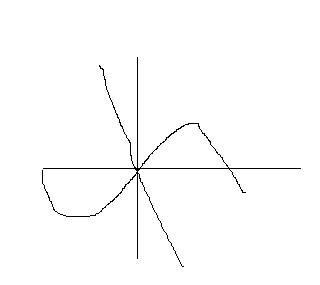# F(x) = sin(x)+2x+1, show (f^(-1))'(1)=1/3

Gold Member

## Homework Statement

If f(x) = sin(x) +2x+1 and g is the inverse function of f, what is the value of g'(1)? Answer is 1/3. No calculator allowed. About 2 minutes allowed to solve.

## Homework Equations

g'(a)=1/f'(g(a)) (from f(g(x))=x, taking derivative of both sides)

## The Attempt at a Solution

Plugging into the above equation, I just get g'(1) =1/(cos(g(1)+2), which looks like a dead end.
I thought of trying the reverse, using f'(1)=1/g'(f(1)), but that just gets me that cos(1)+2=1/g'(3), which is even further from a solution. I also thought that if I used f'(x)=1/g'(f(x)) and found x such that f(x) = 1, that would give me the answer, but then I get the equation sin(x) =-2x , which is not getting me anywhere either for a problem that is supposed to be simple.

Simon Bridge
Homework Helper
If x is in degrees rather than radians, then sin(1) is almost zero.

Gold Member

ehild
Homework Helper
I get the equation sin(x) =-2x , .
Just plot it, you see a solution of that equation. Can the line y=-2x cross the curve z=sin(x) anywhere else but at zero?
Is f(x) monotonous? If it has the value of 1 somewhere can it be the same again somewhere else?

Last edited:
•Gold Member
Thanks for the input, ehild. As you pointed out, "0" plays a key role (which I needed to figure out without actually plotting it: see the conditions in my original post. What's the mathematical equivalent for the physicist's "Gedankenexperiment"?). So, quite simply, since f(0)=1, g(1) = 0, and then 1/(cos(g(1))+2) =1/(cos(0)+2)= 1/3. Finished. Thanks.

Simon Bridge
Homework Helper
Thinking about it: If you wrote down ##\sin(x)+2x+1 = 1## ... you could probably just solve it by inspection.
(sit back and look at it: on the LHS, x has to be chosen to get rid of the first 2 terms... no?)

Under pressure, you may not see it ... but you do have another bit of information: the metadata that it should be solvable quickly because, otherwise, you'd have been given more time. You kinda knew that, I can tell because that is how you tried sorting your methods ... so how to use it...

The fastest way to solve a problem is by guessing. What are good numbers to guess?
... the thing that makes the equation annoying is the sine, but you know values of x that make sin(x) nice ... they are 0, pi/6, pi/2, etc. The ones with pi in them will give you an irrational answer ... so probably not them. But you don't even have to think that hard: just start by guessing the easy one.

That way of thinking depends on how much you trust the problem setter to get it right... one way they could have got it wrong is if there was more than one solution. You can check, as you have seen, by making a quick sketch - knowing the properties of straight lines and the sine function.
But, as you've observed, you need to be practised at this or you'll run out of time.

Note: my first instinct was to try understanding the function by looking at how it behaves at some values ... then move on: but ehild beat me to it ;)
The trick to doing the really fast ones is to get used to how common functions behave: build your intuition.

In a full exam, though, this sort of question is as much about time management as it is about getting the answer right: you are often better off to move on that stick with a problem. When you've got spare time at the end, come back to it. The checking for multiple solutions is something to do at the end.

•ehild
Homework Helper
Thanks for the input, ehild. As you pointed out, "0" plays a key role (which I needed to figure out without actually plotting it: see the conditions in my original post.
I meant a sketch when I said "plot", something like that :)You should know how a sine function looks like and how the function -2x looks like.

•